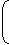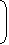# Aptitude - Surds and Indices

### Exercise :: Surds and Indices - General Questions

6.

(256)0.16 x (256)0.09 = ?

 A. 4 B. 16 C. 64 D. 256.25

Explanation:

(256)0.16 x (256)0.09 = (256)(0.16 + 0.09)

= (256)0.25

= (256)(25/100)

= (256)(1/4)

= (44)(1/4)

= 44(1/4)

= 41

= 4

7.

The value of [(10)150 ÷ (10)146]

 A. 1000 B. 10000 C. 100000 D. 106

Explanation:

 (10)150 ÷ (10)146 = 10150 10146

= 10150 - 146

= 104

= 10000.

8.

 1 + 1 + 1 = ? 1 + x(b - a) + x(c - a) 1 + x(a - b) + x(c - b) 1 + x(b - c) + x(a - c)

 A. 0 B. 1 C. xa - b - c D. None of these

Explanation:

Given Exp. =
1  +  1  +  11 + xb + xcxa xa1 + xa + xcxb xb1 + xb + xaxc xc

 = xa + xb + xc (xa + xb + xc) (xa + xb + xc) (xa + xb + xc)

 = (xa + xb + xc) (xa + xb + xc)

= 1.

9.

(25)7.5 x (5)2.5 ÷ (125)1.5 = 5?

 A. 8.5 B. 13 C. 16 D. 17.5 E. None of these

Explanation:

Let (25)7.5 x (5)2.5 ÷ (125)1.5 = 5x.

 Then, (52)7.5 x (5)2.5 = 5x (53)1.55(2 x 7.5) x 52.5 = 5x 5(3 x 1.5)515 x 52.5 = 5x 54.55x = 5(15 + 2.5 - 4.5)5x = 513x = 13.

10.

(0.04)-1.5 = ?

 A. 25 B. 125 C. 250 D. 625

Explanation:

 (0.04)-1.5 =4-1.5 100

 =1-(3/2) 25

= (25)(3/2)

= (52)(3/2)

= (5)2 x (3/2)

= 53

= 125.